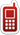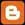MLK Home & Online Tutor+91-836-801-8076+91-999-034-6833Home Tutors available in Ghaziabad, Noida & East Delhi | Ghaziabad- Indirapuram, Vaishali, Vasundhara, Kaushambhi, Shahibabad, Shalimar Garden, Siddharth Vihar, Pratap Vihar, Crossing Republik, Mohan Nagar, Kavi Nagar, Raj Nagar, Shastri Nagar, Nehru Nagar, Patel Nagar, Model Town, Govind Puram, Chiranjeev Vihar, Gandhi Nagar, Lal Kuan, Wave City, Raj Nagar Extension | Noida- Noida(All Sectors), Greater Noida, Noida West(Noida Extension-Gour City-1,2; Amarpali Dream Valley; SuperTech Eco Village) | Delhi- East Delhi(Preet Vihar, Vivek Vihar, Shahdara, Mayur Vihar, Patparganj, Lakshmi Nagar, Shakarpur), South Delhi, West Delhi, North Delhi & Central Delhi.

## AREA WISE CHEMISTRY TUTORSFollow @tutormlk# Math Tutoring for Grade 7- Find Math home Tutors, Online Tutors, home Tuition & Private Tutors for Class VII

Is your child struggling in school? Does your child stuck on math homework?

Or

Are you having difficulty working out math problems? Are you looking out for a grade 7 math home tutor?

MLK Home & Online Tutor can help you find the most qualified home & Online Math tutors for class/grade 7 across Ghaziabad, Noida, Delhi, Bangalore in Karnataka, Pune in Maharashtra, Hyderabad in Andhra Pradesh, Mumbai in Maharashtra, New Delhi in Delhi, Gurgaon in Haryana, Chandigarh in Punjab and Haryana, Noida in Uttar Pradesh, Ahmedabad in Gujarat & Chennai in Tamilnadu.

MLK Home & Online Tutor can help you find Maths tutors for class/grade VII we have available near you. We will send him to your home for one free trial class, if satisfied with his tutoring, then continue. We are the only place, where just a single Call or Message will recommend you Maths tutor class/grade 7 for your personal needs, arranged according to their rates and distance from you. Maths can be an extremely tough subject to get to grips with, but private Maths tuition can really help your learning!

If you are a class/grade VII Maths tutor wishing to provide home tutoring in Maths class/grade 7, or in any of our other subjects, please register with MLK Home & Online Tutor.

Click here for a Home Tutor or an Online Tutor? MLK Home & Online Tutoring Service will assist your child in simplifying concepts and building confidence in approaching math. We serve students from Grade 6-12. In addition to math, tutoring is available in all core subjects-Physics, Chemistry, General science, Social studies test preparation including OLYNPIADS, NTSE exams, SAT, GMAT, GRE, MAT, CAT and foundation course for AIEEE and IIT-JEE.

## Get the Math Help Now!

Getting help is simple. You just tell us what you are working on and we match a professional Home tutor. Our expert, private, professional home tutor helps you solve your math problems step by step until your home work is finished. Home tutor at MLK Home and Online Tutoring Service provides you with worksheet and problem sets to practice your skills.

## Get a Home Tutor for Math Now!

Our home tutor is trained to identify the exact obstacle that may be hindering students' studies. He supplements the course's curriculum to ensure efficient and motivating home tutoring. Our home tutors are flexible in their schedule as they are available seven days a week for In-home tutoring.

## Affordable Math Test Preperation Programs

MLK Home and Online Tutoring Service 's Math Refresher course is designed to build a strong foundation in the basic math skills essential for success on the following tests:

 SAT GMAT GRE CAT MAT IIT-JEE AIEEE OLYMPIADS NDA SSC Bank PO/Clerk RRB Delhi & UP-SI Exam

## Topics Covered by our Math Home Tutoring Program:

 Arithmetic Pre-Algebra Algebra 1 Algebra 2 Linear Algebra Geometry Pre-Calculus Calculus Statistics & Probability Trigonometry General Math

## Math Syllabus For Class VII

### Number System

#### (i) Knowing our Numbers: Integers

• Multiplication and division of integers (through patterns). Division by zero is meaningless
• Properties of integers (including identities for addition & multiplication, commutative, associative, distributive) (through patterns). These would include examples from whole numbers as well. Involve expressing commutative and associative properties in a general form. Construction of counterexamples, including some by children. Counter examples like subtraction is not commutative.
• Word problems including integers (all operations)

#### (ii) Fractions and rational numbers:

• Multiplication of fractions
• Fraction as an operator
• Reciprocal of a fraction
• Division of fractions
• Word problems involving mixed fractions
• Introduction to rational numbers (with representation on number line)
• Operations on rational numbers (all operations)
• Representation of rational number as a decimal.
• Word problems on rational numbers (all operations)
• Multiplication and division of decimal fractions
• Conversion of units (length & mass)
• Word problems (including all operations)

#### (iii) Powers:

• Exponents only natural numbers.
• Laws of exponents (through observing patterns to arrive at generalisation.)

### Algebraic Expressions

• Generate algebraic expressions (simple) involving one or two variables
• Identifying constants, coefficient, powers
• Like and unlike terms, degree of expressions e.g., x2 y etc. (exponent≤ 3, number of variables )
• Addition, subtraction of algebraic expressions (coefficients should be integers).
• Simple linear equations in one variable (in contextual problems) with two operations (avoid complicated coefficients) Ratio and Proportion
• Ratio and proportion (revision)
• Unitary method continued, consolidation, general expression.
• Percentage- an introduction.
• Understanding percentage as a fraction with denominator 100
• Converting fractions and decimals into percentage and vice-versa.
• Application to profit and loss (single transaction only)
• Application to simple interest (time period in complete years).

### Geometry

#### (i) Understanding shapes:

• Pairs of angles (linear, supplementary, complementary, adjacent, vertically opposite) (verification and simple proof of vertically opposite angles)
• Properties of parallel lines with transversal (alternate, corresponding, interior, exterior angles)

#### (ii) Properties of triangles:

• Angle sum property (with notions of proof & verification through paper folding, proofs using property of parallel lines, difference between proof and verification.)
• Exterior angle property
• Sum of two sides of a it’s third side
• Pythagoras Theorem (Verification only)

#### (iii) Symmetry

• Recalling reflection symmetry
• Idea of rotational symmetry, observations of rotational symmetry of 2-D objects. (900, 1200, 1800)
• Operation of rotation through 900 and 1800 of simple figures.
• Examples of figures with both rotation and reflection symmetry (both operations)
• Examples of figures that have reflection and rotation symmetry and vice-versa
• #### (iv) Representing 3-D in 2-D:

• Drawing 3-D figures in 2-D showing hidden faces.
• Identification and counting of vertices, edges, faces, nets (for cubes cuboids, and cylinders, cones).
• Matching pictures with objects (Identifying names)
• Mapping the space around approximately through visual estimation.

#### (v) Congruence

• Congruence through superposition (examplesblades, stamps, etc.)
• Extend congruence to simple geometrical shapes e.g. triangles, circles.
• Criteria of congruence (by verification) SSS, SAS, ASA, RHS

#### (vi) Construction (Using scale, protractor, compass)

• Construction of a line parallel to a given line from a point outside it.(Simple proof as remark with the reasoning of alternate angles)
• Construction of simple triangles. Like given three sides, given a side and two angles on it, given two sides and the angle between them

### Mensuration

• Revision of perimeter,
• Idea of , Circumference of Circle
• Area Concept of measurement using a basic unit area of a square, rectangle, triangle, parallelogram and circle
• Area between two rectangles and two concentric circles.

### Data handling

(i) Collection and organisation of data – choosing the data to collect for a hypothesis testing.
(ii) Mean, median and mode of ungrouped data – understanding what they represent.
(iii) Constructing bargraphs (iv) Feel of probability using data through experiments. Notion of chance in events like tossing coins, dice etc. Tabulating and counting occurrences of 1 through 6 in a number of throws. Comparing the observation with that for a coin.Observing strings of throws, notion of randomnessFollow @tutormlkFollow @tutormlk